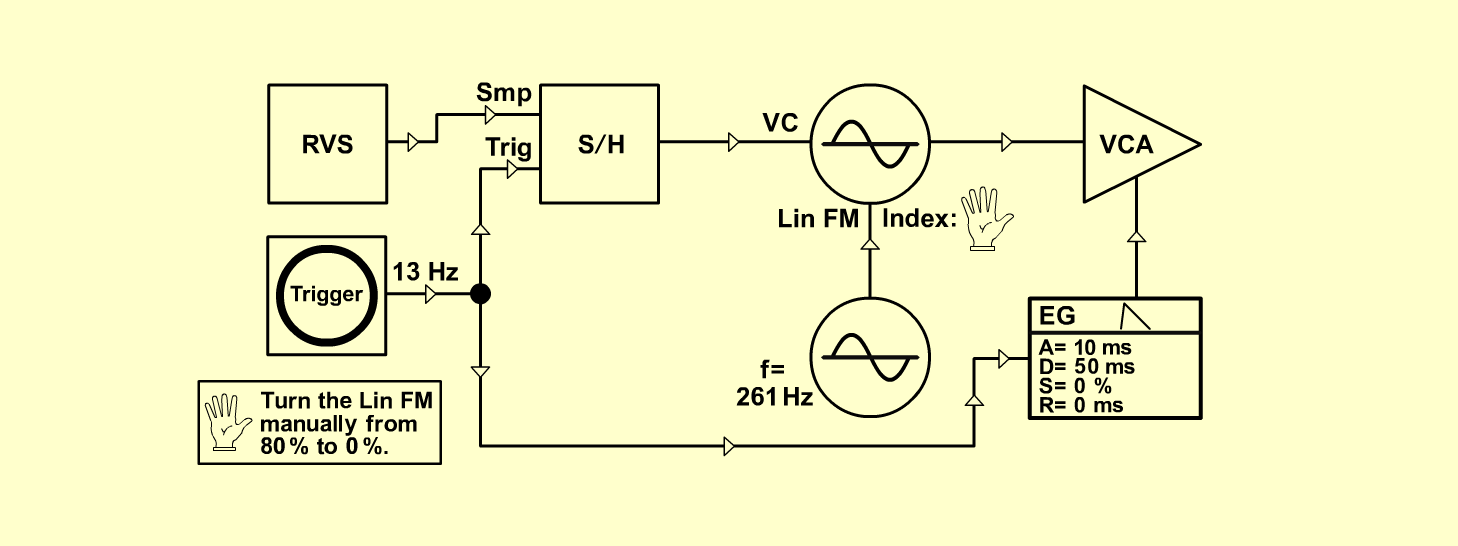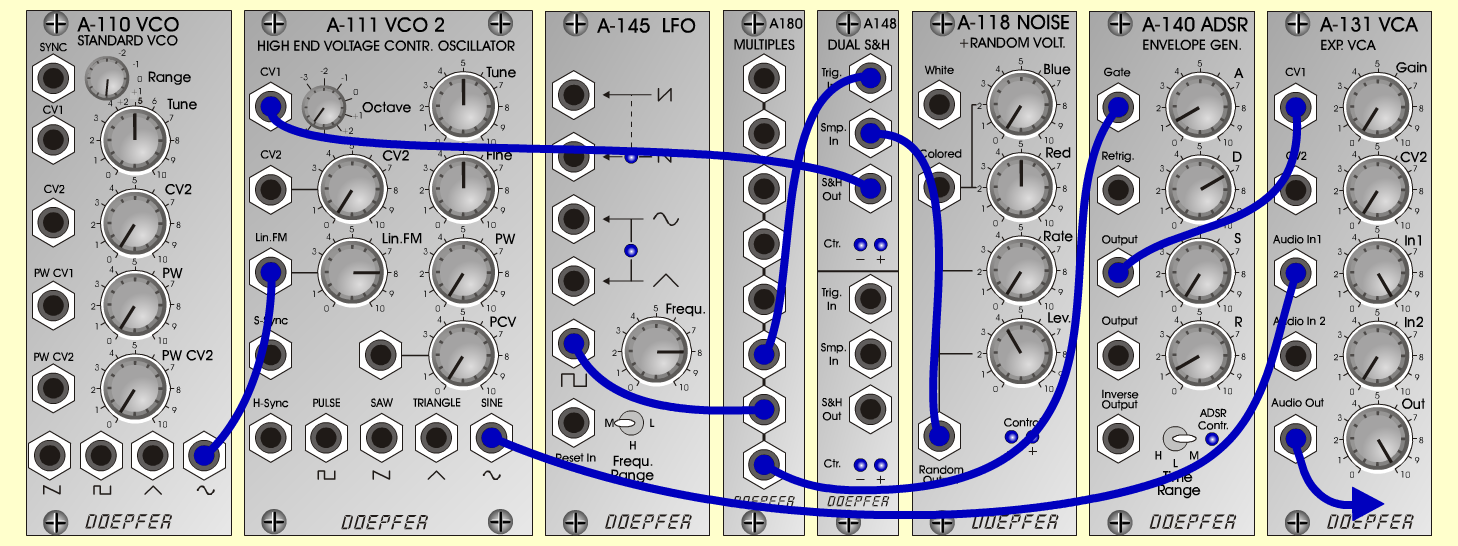SimpleLinFMTextureD o e p f e r A - 100 Connections: Settings: A-110 (Sine)  <=>  A-111 (Lin FM)   A-111 (Sine)  <=>  A-131 (Audio In)   A-118 (Random)  <=>  A-148 (Smp In)   A-145 (Square)  <=>  A-180   A-180  <=>  A-148 (Trig In)   A-148 (S&H Out)  <=>  A-111 (CV1)   A-180 <=>  A-140 (Gate)   A-140 (Output)  <=>  A-131 (CV 1)   A-131 (Audio Out)  <=> Amplifier A-110 (Range = +3, Tune = 5)   A-111 (Oct = +4, Tune = 5, Fine = 5,   Lin FM = 8 ~ 3)   A-118 (Blue = 0, Red = 5, Rate = 0, Level = 4)   A-145 (Frq = 8, Range = M)   A-140 (A = 1, D = 7, S = 0, R = 1, Range = M)   A-131 (Gain = 0, Audio In 1 = 10,  Audio Out = 10) Notes: Turn the Lin-FM-Pot (A-111) manually    from 8 to 0. Josef MuellerSound sample SimpleLinFM